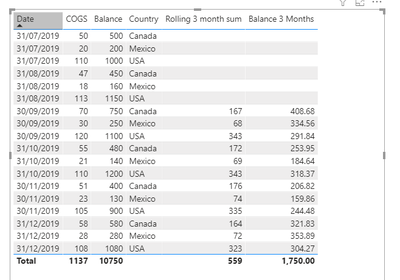cancel
Showing results for
Did you mean:

Fabric is Generally Available. Browse Fabric Presentations. Work towards your Fabric certification with the Cloud Skills Challenge.Helper III

## Rolling 3 month sum, divide by value across tables

Hello,

My problem is a bit difficult to explain in words alone, so here's a spreadsheet to make it clearer:I have 2 fact tables. Columns with black text are columns that I have, and columns in blue are ones that I don't have.
The only number I care about obtaining is the one on the far right (in blue). My dataset is small so creating calculated columns isn't a problem if necessary.

I have created a rolling 3 month sum measure as follows:

``````Rolling 3 month sum =
CALCULATE(
SUM(Table1[COGS]),
DATESINPERIOD(Table1[Date],
LASTDATE(Table1[Date]), -3, MONTH)
)``````

but when dividing 'Table2'[Balance] by the result in this measure, it needs to divide it by country, not as a whole.

Any help is appreciated as always 🙂

1 ACCEPTED SOLUTIONSuper User

Hi @DCELL ,

I created a model with the following tables:

• COGS
• BALANCE
• Country (Dimension table)
• Date (Dimension table)

The dimension table are use to make a relationship between the COGS and Balance table then create the following two measures:

``````Rolling 3 month sum =
IF (
DATEDIFF (
MINX (
DATESINPERIOD ( 'Calendar'[Date]; LASTDATE ( 'Calendar'[Date] ); -3; MONTH );
'Calendar'[Date]
);
MAXX (
DATESINPERIOD ( 'Calendar'[Date]; LASTDATE ( 'Calendar'[Date] ); -3; MONTH );
'Calendar'[Date]
);
DAY
) < 61;
BLANK ();
CALCULATE (
SUM ( COGS[COGS] );
DATESINPERIOD ( 'Calendar'[Date]; LASTDATE ( 'Calendar'[Date] ); -3; MONTH )
)
)

Balance 3 Months =
IF (
[Rolling 3 month sum] = BLANK ();
BLANK ();
SUM ( Balance[Balance] ) / [Rolling 3 month sum] * 91
)``````

See result below and in attach file:Regards

Miguel Félix

Proud to be a Super User!

Check out my blog: Power BI em Português2 REPLIES 2Super User

Hi @DCELL ,

I created a model with the following tables:

• COGS
• BALANCE
• Country (Dimension table)
• Date (Dimension table)

The dimension table are use to make a relationship between the COGS and Balance table then create the following two measures:

``````Rolling 3 month sum =
IF (
DATEDIFF (
MINX (
DATESINPERIOD ( 'Calendar'[Date]; LASTDATE ( 'Calendar'[Date] ); -3; MONTH );
'Calendar'[Date]
);
MAXX (
DATESINPERIOD ( 'Calendar'[Date]; LASTDATE ( 'Calendar'[Date] ); -3; MONTH );
'Calendar'[Date]
);
DAY
) < 61;
BLANK ();
CALCULATE (
SUM ( COGS[COGS] );
DATESINPERIOD ( 'Calendar'[Date]; LASTDATE ( 'Calendar'[Date] ); -3; MONTH )
)
)

Balance 3 Months =
IF (
[Rolling 3 month sum] = BLANK ();
BLANK ();
SUM ( Balance[Balance] ) / [Rolling 3 month sum] * 91
)``````

See result below and in attach file:Regards

Miguel Félix

Proud to be a Super User!

Check out my blog: Power BI em PortuguêsHelper III

Hi @MFelix ,

Thank you so much for your help. It works!Announcements#### Power BI Monthly Update - November 2023

Check out the November 2023 Power BI update to learn about new features.#### Fabric Community News unified experience

Read the latest Fabric Community announcements, including updates on Power BI, Synapse, Data Factory and Data Activator.#### The largest Power BI and Fabric virtual conference

130+ sessions, 130+ speakers, Product managers, MVPs, and experts. All about Power BI and Fabric. Attend online or watch the recordings.Top Solution Authors
Top Kudoed Authors
Users online (3,924)